Note: This is an archvied version of our old webpage. Some links might be broken. The current one can be found here.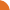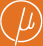Chair for Foundations of Software Reliability and Theoretical Computer Science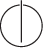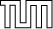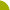Publications - Approximative Methods for Monotone Systems of min-max-Polynomial EquationsReference:

Javier Esparza, Thomas Gawlitza, Stefan Kiefer, and Helmut Seidl. Approximative methods for monotone systems of min-max-polynomial equations. Technical report, Technische Universität München, Institut für Informatik, February 2008.

Abstract:

A monotone system of min-max-polynomial equations (min-max-MSPE) over the variables X_1, ..., X_n has for every i exactly one equation of the form X_i = f_i(X_1, ..., X_n) where each f_i(X_1, ..., X_n) is an expression built up from polynomials with non-negative coefficients, minimum- and maximum-operators. The question of computing least solutions of min-max-MSPEs arises naturally in the analysis of recursive stochastic games. Min-max-MSPEs generalize MSPEs for which convergence speed results of Newton's method have been established in previous papers. We present the first methods for approximatively computing least solutions of min-max-MSPEs which converge at least linearly. Whereas the first one converges faster, a single step of the second method is cheaper. Furthermore, we compute epsilon-optimal positional strategies for the player who wants to maximize the outcome in a recursive stochastic game.

Suggested BibTeX entry:

@techreport{EGKS08:minMaxTechRep,
author = {Javier Esparza and Thomas Gawlitza and Stefan Kiefer and Helmut Seidl},
institution = {Technische Universit\"{a}t M\"{u}nchen, Institut f\"{u}r Informatik},
month = {February},
title = {Approximative Methods for Monotone Systems of min-max-Polynomial Equations},
year = {2008}
}GZipped PostScript (181 kB)PDF (267 kB)Conference version